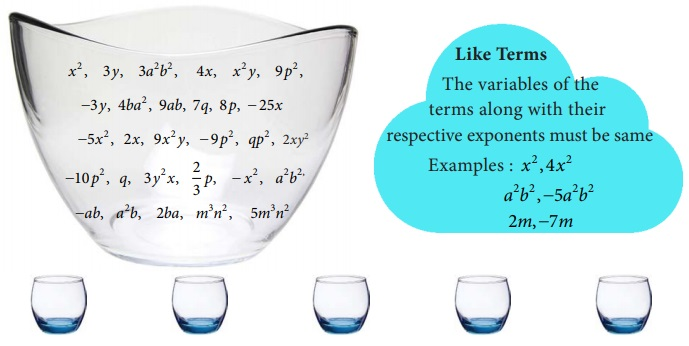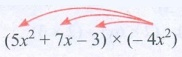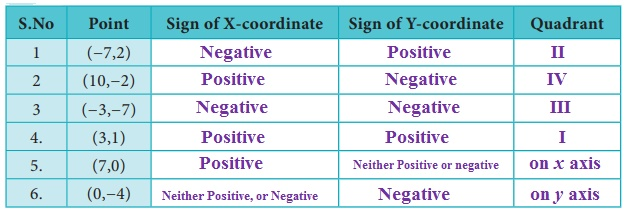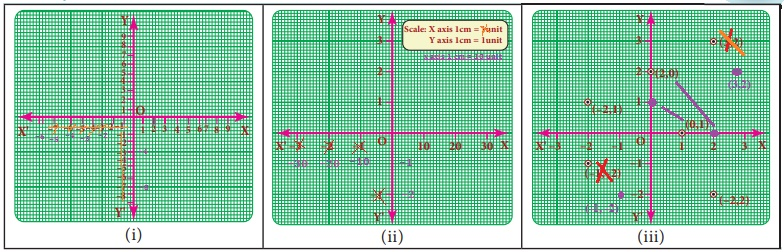Home | | Maths 8th Std | Try these, Recap Exercise, Student Activities, Think and answer

# Try these, Recap Exercise, Student Activities, Think and answer

8th Maths : Chapter 3 : Algebra : Try these, Recap Exercise, Student Activities, Think and answer - Numerical Example solved problems and Numerical problems Questions with Solution

Recap Exercise

1. Write the number of terms in the following expressions

(i) x + y + z  xyz [Answer: 4 terms]

(iii) a2b2c − ab2c2 + a2bc2 + 3abc [Answer: 4 terms]

(iv) 8x2 − 4xy + 7xy[Answer: 3 terms]

2. Identify the numerical co-efficient of each term in the following expressions.

(i) 2x2  5xy + 6 y2 + 7x 10 y + 9

(ii) x/3 + 2y/5  xy + 7

(i) 2x2 − 5xy + 6y2 + 7x − 10y + 9

Numerical co efficient in 2x2 is 2

Numerical co efficient in −5xy is −5

Numerical co efficient in 6y2 is 6

Numerical co efficient in 7x is 7

Numerical co efficient in −10y is − 10

Numerical co−efficient in 9 is 9

(ii) x/3 + 2y/5 − xy + 7

Numerical co efficient in x/3 is 1/3

Numerical co efficient in 2y/5 is 2/5

Numerical co efficient in −xy is −1

Numerical co efficient in 7 is 7

3. Pick out the like terms from the following:Solution:4. Add : 2x , 6 y, 9x  2y

Solution:

2x + 6+ 9x − 2y = 2x + 9x + 6y − 2y = (2 + 9)x + (6 − 2)y = 11x + 4y

5. Simplify : (5x 3 y3  3x 2 y2 + xy + 7) + (2xy + x 3 y3  5 + 2x 2 y2 )

Solution:

(5x3y3 − 3x2y2 + xy + 7) + (2xy + x3y3 − 5 + 2x2y2)

= 5x3y3 + x3y3 – 3x2y2 + 2x2y2 + xy + 2xy + 7 − 5

= (5 + 1) x3y3 + (−3 + 2) x2y2 + (1 + 2) xy + 2

= 6 x3y3 − x2y2 + 3xy + 2

6. The sides of a triangle are 2x  5y + 9, 3y + 6x  7 and x + y + 10 . Find the perimeter of the triangle .

Solution:

Perimeter of the triangle = Sum of three sides

= (2x − 5y + 9) + (3y + 6x −7) + (−4x + + 10)

= 2x − 5y + 9 + 3y + 6x − 7 − 4x + y + 10

= 2x + 6x − 4x − 5y + 3y + y + 9 − 7 + 10

= (2 + 6 − 4)x + (−5 + 3 + l) y + (9 − 7 + 10)

= 4x –y + 12

Perimeter of the triangle = 4x –y + 12 units.

7. Subtract −2mn from 6mn.

Solution:

mn − (−2 mn) = 6 mn + (+2 mn)

= (6 + 2) mn = 8 mn

8. Subtract 6a 2  5ab + 3b2 from 4a 2  3ab + b2 .

Solution:

(4a2 − 3ab + b2) − (6a2 − 5ab + 3b2)

= (4a2 − 6a2) + (− 3ab − (−5 ab)] + (b2 − 3b2)

= (4 − 6) a2 + [−3ab + (+5 ab)] + (1 − 3) b2

= [4 + (− 6)] a2 + (−3 + 5) ab + [1+ (−3)] b2

= −2a2 + 2ab − 2b2

9. The length of a log is 3a + 4b  2 and a piece (2a  b) is removed from it. What is the length of the remaining log?Solution:

Length of the log = 3a + 4b − 2

Length of the piece removed = 2a − b

Remaining length of the log = (3a + 4b −2) − (2a − b)

= (3a – 2a) + [4b − (− b)] − 2

= (3 − 2)a + (4 + 1)b − 2

a + 5b – 2

10. A tin had x litres of oil. Another tin had (3x2 + 6x  5) litres of oil.

The shopkeeper added (x+7) litres more to the second tin. Later, he sold (x2+6) litres of oil from the second tin. How much oil was left in the second tin?

Solution:

Quantity of oil in the second tin = 3x2 + 6x − 5 litres.

Quantity of oil added = x + 7 litres

Total quantity of oil in the second tin

= (3x+ 6x − 5) + (x + 7) litres

= 3x2 + (6x + x) + (−5 + 7) = 3x2 + (6 + 1 )x + 2

= 3x2 + 7x + 2 litres

Quantity of oil sold = x2 + 6 litres

Quantity of oil left in the second tin

= (3x+ 7+ 2) − (x2 + 6) = (3x2 − x2) + 7x + (2 − 6)

= (3 − 1) x2 + 7x + (−4) = 2x2 + 7x − 4

Quantity of oil left = 2x2 + 7x − 4 litres

Think

Every algebraic expression is a polynomial. Is this statement true? Why?

Solution:

No, This statement is not true. Because Polynomials contain only whole numbers as the powers of their variables. But an algebraic expression may contains fractions and negative powers on their variables.

Eg. 2y2 + 5y−1 − 3 is a an algebraic expression. But not a polynomial.

Try these

Find the product of

(i) 3ab2 , 2a2b3

(ii) 4xy, 5 y2 x,(x2 )

(iii) 2m5n3p

Solution:

(i) (3ab2) × (−2a2b3= (+) × (−) × (3 × 2) × (a × a2) × (b2 × b3) = − 6a3 b5

(ii) (4xy) × (5y2x) × (−x2) = (+) × (+) × (−) × (4 × 5 × 1) × (x × x × x2) × (y × y2)

= −20 x4y3

(iii) (2m) × (−5n) × (−3p) = (+) × (−) × (−) × (2 × 5 × 3) × m × n × p

= + 30 mnp = 30 mnp

Think

Why 3+(4x–7y) ≠ 12x−21y ?

Solution:

Addition and multiplication are different 3 + (4x − 7y) = 3 + 4x − 7y

We can add only like terms.

Try these

Multiply

(i) (5 x2 + 7– 3) by –4x2

(ii) (10– 7+ 5z) by 6xyz

(ii) (ab+3bc –5ca) by 3a2bc

(iv) (4m2 – 3m + 7) by –5m3

Solution:

(i) (5x2 + 7x − 3) by – 4x2(5x2 + 7x − 3) × (– 4x2) = 5x2 (− 4x2) + 7x (− 4x2) − 3(− 4x2)

= − 20 x4 − 28x3 + 12x2

(ii) (10x −7y + 5z) by 6xyz(10x −7y + 5z) × 6xyz = 6xyz (10x − 7y + 5z[ Multiplication is commutative]

= 6xyz(10x) + 6xyz(−7y) + 6xyz(5z)

= (6 × 10) (x × x × y × z) + (6 × −7) + (x × y × y × z ) + (6 × 5)(x × y × z × z)

= 60x2yz + (−42xy2z) + 30xyz2

= 60x2yz − 42xy2z + 30xyz2

(iii) (ab + 3bc − 5ca) by 3a2 bc

(ab + 3bc − 5ca) × (3a2bc) = ab(3a2bc) + 3bc (3a2bc) − 5ca (3a2bc)

= 3a2b2c + 9a2b2c2 − 15a3bc2

(iv) (4m2 − 3+ 7) by − 5m3

(4m2 − 3m + 7) × (− 5m3) = 4m2 (− 5m3) − (3m) (− 5m3) + 7(− 5m3)

= − 20m5 + 15m4 − 35m3

Try these

Multiply

(i) (a − 5) and (a + 4)

(ii) (a + b) and (a − b)

(iii) (m4 + n4 ) and (m − n)

(iv) (2+ 3)(+ 4)

(v) (3+ 7)( x −5)

(vi) (− 2)(6− 3)

Solution:

(i) (a − 5) and (a + 4)

(a − 5) (a + 4) = a(a + 4) − 5(a + 4)

= (a × a) + (a × 4) + (−5 × a) + (−5 × 4)

a2 + 4a − 5a − 20 = a2 − a − 20

(ii) (a + b) and (a − b)

(a + b) (a − b) = a(a − b) + b(a − b)

= (a × a) + (a × −b) + (b × a ) + b(− b)

a2 − ab + ab − b2 = a2 − b2

(iii) (mn4) and (m − n)

(m4 + n4) (m − n) = m4 (m − n) + n4 (m − n)

= (m4 × m) + (m4 × (− n)) + (n4 × m) + (n4 × (−n))

m5 − m4 n + mn4 – n5

(iv) (2x + 3) (x + 4)

(2x + 3) (x + 4)  = 2x(x + 4) + 3(x + 4)

= (2x2 × x) + (2x × 4) + (3 × x) + (3 × 4)

= 2x2 + 8x + 3x + 12 = 2x2 + 11x + 12

(v) (3x + 7) (x − 5)

(3x + 7) (x − 5)  = x(3x + 7) − 5(3x + 7)

= (x × 3x) + (x × 7) + (−5 × 3x) + (−5 × 7)

= 3x2 + 7x − 15x − 35

= 3x2 − 8x – 35

(vi) (x − 2) (6x − 3)

(x − 2) (6x − 3)  = x(6x − 3) − 2(6x − 3)

= (x × 6x) + (x × (−3) − (2 × 6x) − (2 × 3)

= 6x2 − 3x − 12x + 6

= 6x2 − 15x + 6

Think

(i) In 3x2 (x4–7x3+2), what is the highest power in the expression?

Solution:

3x2 (x4 − 7x3 + 2)  = (3x2) (x4) + 3x(− 7x3) +  (3x2)2

= 3x − 21x5 + 6x2

Highest power is 6 in x6.

(ii) Is –5y2 +2y–6 = –(5y2 +2y–6)? If not, correct the mistake.

Solution:

No, – 5y2 + 2y – 6 = − (5y2 − 2y + 6 )

Think

Are the following correct?

(i) x3/x8 = x8-3 = x5

(ii) 10m4 / 10m= 0

(iii) When a monomial is divided by itself, we will get 1?

Solution:

(i) x3 / x8 = x8 – 3 = x5

x3 / x8 = x3 – 8 = x5 (or) x3 / x8 = 1 / x8 – 3 = 1 / x5

(ii) 10m4 / 10m4 = 0

10m4 / 10m4 = [10/10] m4−4 = 1 m0 = 1     [ ∵ m0 = 1]

The given answer is not correct

(iii) When a monomial is divided by itself, we will get 1?

When a monomial is divided by itself, we will get 1.

Eg. x/x = x1 – 1 = x0 = 1

The given statement is correct

Try these

Divide

(i) 12x 3y2 by x2(ii) 20a5b2 by 2a3b7 (iii) 28a4 c2 by 21ca2

(iv) (3 x 2 y)3 6x2y(v) 64m4 (n2)3 ÷ 4m2n(vi) (8 x 2 y 2 )3 ÷ (8x 2 y2 )2

(vii) 81 p2q4 ÷ √[81p2q]

(vii) ( 4x2 y3 )0 ÷ [( x3)2]/x6

Solution:Solution:

(i) 12x3y2 / x2y = 12x3−2 y2−1  = 12x1y1 = 12 xy

(ii) −20a5b2 / 2a3b7 = −20a5 − 3 / 2b7 − 2  = −10a2 / b5

(iii) 28 a4c2 / 21ca2 = [28 / 21] a4 – 2 c2 – 1 = 4/3 a2c1 = 4/3 a2c

(iv) (3x2y)3 / 6x2y3 = 27 x6y3 / 6 x2y = [9/2] x6–2 = 9/2 x4

(v) 64m4(n2)3 / 4m2n2 = 64m4n6 / 4m2n2 = 16 m4−2 n6−2 = 16 m2n4

(vi) (8x2y2)3 / (8x2y2)2 = 512 x6y6 / 64 x4y4

= 8x6−4 y6−4

= 8x2y2

(vii) 81p2q4 / √81p2q4 = 81p2q4 / 9pq2 = 9 p2−1q4–2 = 9 pq2

(viii) (4x2y3)0 /  [ (x3)2 / x6 ] = 1 / [ x6 / x6 ] = 1 / 1 = 1

Think

Are the following divisions correct?Solution:

(i) [4y + 3] / 4 = y + 3[4y + 3] / 4 = [4/ 4] + [3 / 4] = y + [3/4] is the correct answer.

The given answer is not correct.

(ii) [5m2 + 9] / 9 = 5m2[5m2 + 9] / 9 = [5m2 / 9] + [9 / 9] = [ 5/9 m2 ] + 1 is the correct answer

The given answer is not correct.

(iii) [2x2 + 8] / 4 = 2x2 + 2 If not, correct it.[2x2 + 8] / 4 = [(2x2) / 4 ] + [ 8 / 4 ] = (1/2) x2 + 2 is the correct answer

The given answer is not correct.

Try these

(i) (16 y5  8y2 ) ÷ 4 y

(ii) ( p5 q2 + 24 p3 q 128q3 ) ÷ 6q

(iii) (4m2n + 9n2m + 3mn) ÷ 4mn

Solution:(i) (16y− 8y2) ÷ 4y

(16y− 8y2) / 4y = [16y5 / 4]− [ 8y/ 4y ] = 4y5 – 1 − 2y2 – 1 = 4y4 – 2y

(ii) ( p5q+ 24p3q − 128 q3 ) ÷ 6q

p5q+ 24p3q − 128 q3 ) / 6q  = [ p5q/ 6] + [ 24p3q / 6] – [ 128q3 / 6]

= (1/6) p5q2 – 1 + 4p3q1 – 1 – (64/3)q3 – 1

= (1/6)p5q+ 4p3q0 – (64/3)q2 = (1/6)p5q + 4p3 – (64/3)q2

(iii) ( 4m2n + 9n2m + 3mn ) ÷ 4mn

[ 4m2n + 9n2 m + 3mn ] / 4mn = [ 4m2n / 4mn ] + [ 9n2m / 4mn ] + [ 3mn / 4mn ]

m2−1n1−1 + (9/4) m1−1n2−1 + (3/4)m1−1n1−1

m1n0 + (9/4)m0 n1 + (3/4)m0n= m + (9/4)n + 3/4.    ∵ n=1]

Try these

Expand the following

(i) ( p + 2)2 =  p2 + 2(p) (2) + 22 =  p2 + 4p + 4

(ii) (3 − a)2 =  32 − 2(3) (a) + a2 = 9 − 6a + a2

(iii) (62 − x2 ) = (6 + x) (6 − x)

(iv) (a + b)2 − (a − b)2 = a2 + 2ab + b2 − (a2 − 2ab + b2)

a2 + 2ab + b2 − a2 + 2ab − b2

= (1 − 1) a2 + (2 + 2) ab + (+1 −1) b2 = 4ab

(v) (a + b)2 = (a + b) × (a + b)

(vi) (m + n)(m − n= m2 − n2

(vii) (m + 7)2 = m2 + 14m + 49

(xiii) (k2 - 49) = (k+ )(k - )

(ix) m2 − 6m + 9 = (m − 3)2

(x) (m − 10)(m + 5) = m2 + (−10 + 5)m + (−10)(5) = m2 − 5m − 50

Think

Which is corrcet? (3a)2 is equal to

(i) 3a2 (ii) 32(iii) 6a2 (iv) 9a2

Solution: (3a)2 = 32a2 = 9a2

Try these

Expand using appropriate identities.

(i) (3 p + 2q)2

(ii) (105)2

(iii) (2x  5d)2

(iv) (98)2

(v) ( y  5)( y + 5)

(vi) (3x)2  52

(vii) (2m + n)(2m + p)

(viii) 203 ×197

(ix) Find the area of the square whose side is (x  2) units.

(x) Find the area of the rectangle whose length and breadth are ( y + 4) units and ( y  3) units.

Solution:

1) (3p + 2q)2

Comparing (3p + 2q)with (a + b)2, we get a = 3p and b = 2q.

(a + b)2 = a2 + 2ab + b2

(3p + 2q)2 = (3p)2 + 2(3p) (2q) + (2q2) = 9p2 + 12pq + 4q2

2) (105)2 = (100+ 5)2

Comparing (100 + 5)2 with (a + b)2, we get a = 100 and b = 5.

(a + b)2 = a2 + 2ab + b2

(100 + 5)2 = (100)2 + 2 (100) (5) + 52 = 10000+ 1000 + 25

1052 = 11,025

3. (2x − 5d)2

Comparing with (a – b)2, we get a = 2x  b = 5d.

(a − b)2 = a2 − 2ab + b2

(2x − 5d)2 = (2x)2 − 2 (2x) (5d) + (5d)2

= 22x2 − 20xd + 52d2 = 4x2 − 20xd + 25d2

4. (98)2 = (100 − 2)2

Comparing (100− 2)2 with (a − b)2 we get

a = 100, b = 2

(a − b)2 = a2 − 2ab + b2

(100 − 2)2 = 1002 − 2 (100) (2) + 22

= 10000 − 400 + 4 = 9600 + 4 = 9604

5. (y − 5) (y + 5)

Comparing (y − 5) (y + 5) with (a − b) (a + b) we get

a = y ; b = 5

(a − b) (a + b) = a2 − b2

(y − 5)(y + 5) = y 52 = y2 – 25

6. (3x)2 − 52

Comparing (3x)2 − 52 with a2 − b2 we have

a = 3x ; b = 5

(a b2) = (a + b) (a − b)

(3x)2 − 52 = (3x + 5) (3x − 5) = 3x (3x − 5) + 5 (3x − 5)

= (3x) (3x) − (3x) (5) + 5 (3x) − 5 (5)

= 9x2 − 15x + 15x − 25 = 9x2 – 25

7. (2m + n) (2m + p)

Comparing (2m + n) (2m + p) with (x + a) (x + b) we have

x = 2n ; a = n ; b = p

(x − a)(x + b) = x2 + (a + bx + ab

(2m + n) (2m + p) = (2m2) + (n + p)(2m) + (n) (p)

= 22m2 + n (2m) + p (2m) + np

= 4m2 + 2mn + 2mp + np

8. 203 × 197 = (200 + 3) (200 − 3)

Comparing (a + b) (a − b) we have

a = 200, b = 3

(a + b) (a − b) =  a2 − b2

(200 + 3) (200 − 3) = 2002 − 32

203 × 197 = 40000 − 9

203 × 197 = 39991

9. Side of a square = x − 2

∴ Area = Side × Side

= (x − 2) (x − 2) = x (x − 2) −2 (x − 2)

x(x) + (x) (−2) + (−2)(x) + (−2) (−2)

x2 − 2x − 2x + 4

x2 − 4x + 4 units square

10. Length of the rectangle = y + 4

breadth of the rectangle = y − 3

Area of the rectangle = length × breadth

= (y + 4)(y − 3) = y+ (4 + (−3)) y + (4) (−3)

yy – 12

Activity

You can visualize the geometrical proof of (a+b)3 with the help of your teacher.

Try these

Expand : (i) ( x + 5)3 (ii) ( y  2)3 (iii) (x + 1)(x + 4)(x + 6)

Solution:

(i) Comparing (x + 5)3 with (a + b)3, we have a = x and b = 4.

(a + b)3 = a3 + 3a2 b + 3ab2 + b3

(x + 5)3 = x3 + 3x2 (5) + 3 (x) (5)2 + 53

. = x3 + 15x2 + 75+ 125

(ii) Comparing (y − 2)3 with (a − b)3 we have a = y b = z

(a − b)3 = a3 − 3a2 b + 3ab2 − b3

(y − 2)2 = y3 – 3y2(2) + 3y(2)2 + 23

y3 − 6y2 + 12y + 8

(iii) Comparing (x + 1) (x + 4) (x + 6) with (x + a) (x + b) (x + c) we have

a = 1 b =  4 and c = 6

(x + a) (x + b) (x + c) = x3 + (a + b + cx2 + (ab + bc + caabc

x3 + (1 + 4 + 6) x2 + ((1) (4) + (4) (6) + (6) (l))x + (1) (4) (6)

x3 + 11x2 + (4 + 24 + 6) x +24

x3 + 11x2 + 34x + 24

Try these

Find the factorsThink

x2–4(x–2) = (x2–4)(x–2)

Is this correct? If not correct it.

Solution:

Not correct

(3a)2 = 32a2 − 9a2

x2 − 4(x − 2) = x2 – 4x + 8

Try these

Factorise the following :

1) 3y + 6

2) 10x 2 +15y

3) 7m(m  5) + 1(5  m

4) 64  x2

5) x2–3x+2

6) y2–4y–32

7) p2+2p–15

8) m2+14m+48

9) x2x–90

10) 9x2–6x–8

Solution:

1. 3y + 6

3y + 6 = 3 × y + 2 × 3

Taking out the common factor 3 from each term we get 3 (y + 2)

∴ 3y + 6 = 3(y + 2)

2. 10x2 + 15y2

10x2 + 15y= (2 × 5 × x × x) + (3 × 5 × y × y)

Taking out the common factor 5 we have

10x2 + 15y2  = 5(2x2 + 3y2)

3. 7m (m − 5) + (15 – m)

7m (m − 5) + (15 – m) = 7 m (m − 5) + (−1 )(−5 + m)

= 7m (m − 5) − 1(m − 5)

Taking out the common binomial factor (m − 5) = (m − 5) (7 m − 1)

4. 64 − x2

64 − x= 82 − x2

This is of the form a2 − b2

Comparing with a2 − bwe have a = 8, b = x

a2− b2 = (a + b) (a − b)

64 − x2 = (8 + x) (8 − x)

5. x2 – 3x + 2x2 – 3x + 2 = x2 − 2x − x + 2

x (x − 2) − (x − 2)

= (x − 2) (x − l)

6. y2 – 4y – 32y2 – 4y – 32 y2 – 8y + 4y − 32

y(y − 8) + 4(y − 8)

= (y − 8) (y + 4)

7. p2 + 2p – 15p2 + 2p – 15 =  p2 + 5p −3p − 15

p(p + 5) −3 (p + 5)

= (p + 5) (p − 3)

8. m2 + 14 m + 48m2 + 14 m + 48 = m2 + 8 m + 6 m + 48

m (m + 8) + 6 (m + 8)

= (m + 6) (m + 8)

9. x2 − x – 90x2 − x – 90 x2 − 10x + 9x − 90

x (x − 10) + 9 (x − 10)

= (x + 9) (x − 10)

10. 9x2 − 6x – 89x2 − 6x – 8 = 9x2 − 12x + 6x − 8

= 3x (3x − 4) + 2(3x − 4)

= (3x + 2) (3x − 4)

Try these

Identify which among the following are linear equations.

(i) 2 + x = 19 − Linear as degree of the variable x is 1

(ii) 7x2 – 5 = 3 − not linear as highest degree of x is 2

(iii) 4p3 = 12 − not linear as highest degree of p is 3

(iv) 6m+2 − Linear, but not an equation

(v) n=10 −  Linear equation as degree of n is 1

(vi) 7k – 12= 0 − Linear equation as degree of k is 1

(vii) 6x/8 + y = 1 − Linear equation as degree of x & y is 1

(viii) 5 + y = 3x − Linear equation as degree of y & x is 1

(ix) 10p+2q=3 − Linear equation as degree of p & q is 1

(x) x2–2x–4 − not linear equation as highest degree of x is 2

Think

(i) Is t(t – 5)=10 a linear e quation? Why?

Solution:

t(t − 5) = 10

= (t × t) – (5 × t) = 10

= t2 − 5t = 10

This is not a linear equation as the highest degree of the variable ‘t’ is 2

(ii) Is x2 =2x , a linear equation? Why?

Solution:

x2 = 2x

x2 − 2x = 0

This is not a linear equations as the highest degree of the variable ‘x’ is 2

Try these

Convert the following statements into linear equations:

• On subtracting 8 from the product of 5 and a number, I get 32.

Solution:

Convert to linear equations:

Given that on subtracting 8 from product of 5 and a, we get 32

5 × x − 8 = 32

5x − 8 = 32

• The sum of three consecutive integers is 78.

Solution:

Sum of 3 consecutive integers is 78

Let 1st integer be ‘x

x + (x + 1) + (x + 2) = 78

x + x + l + x + 2 = 78

3x + 3 = 78

• Peter had a Two hundred rupee note. After buying 7 copies of a book he was left with 60.

Solution:

Let cost of one book be ‘x

∴ Given that 200 −7 × x = 60

∴ 200 − 7x = 60

• The base angles of an isosceles triangle are equal and the verteangle measures 80°.

Solution:

Let base angles each be equal to x & vertex bottom angle is 80°. Applying triangle property, sum of all angles is 180°

∴  x + x + 80 = 180°

∴  2x + 80 = 180

• In a triangle ABC, A is 10o more than B. Also C is three times A. Express the equation in terms of angle B.

Solution:

Let B  = b

Given A = 10° + ∠B = 10 + b

Also given that C = 3 × ∠A = 3 × (10 + b) = 30 + 3b

Sum of the angles = 180°

A + B + C = 180°

10 + b + b + 30 + 3b = 180°

∴  5b + 40 = 180°

Think

Can you get more than one solution for a linear equation?

Solution:

Yes, we can get. Consider the below line or equation

x + y = 5

here, when x = 1, y = 4

when x = 2, y = 3

= 3, y = 2

= 4, y = 1

Hence, we get multiple solutions for the same linear equation.

Try these

1. Solve for ‘x’ and ‘y’

(i) 2x = 10

(ii) 3 + x = 5

(iii) x – 6 = 10

(iv) 3x + 5 = 2

(v) 2x/7 = 3

(vi) –2 = 4m – 6

(vii) 4(3x – 1) = 80

(viii) 3x – 8 = 7 – 2x

(ix) 7 – y = 3(5 – y)

(x) 4(1 – 2y)–2(3 – y) = 0

Solution:

(i) 2x = 10

⇒ x = 10 / 2 = 5

(ii) 3 + x = 5

3 + x = 5

x = 5 – 3 = 2

(iii) x − 6 = 10

x − 6 = 10

x = 10 + 6 = 16

(iv) 3x + 5 = 2

3x + 5 = 2

3x = 2 – 5 = − 3

x = −3/3 = −1

(v) 2x/7  = 3

2x = 3 × 7 = 21

x = 21/2

(vi) − 2x = 4m − 6

− 2x = 4m − 6

−2 + 6 = 4m

4 = 4m

m = 4 / 4 = 1

(vii) 4(3x − 1) = 80

4(3x − l) = 80

12x − 4 = 80

12x = 80 + 4 = 84

x = 84 / 12 = 7

(viii) 3x − 8 = 7 − 2x

3x − 8 =  7 − 2x

3x + 2x = 7 + 8 = 15

5x = 15

x = 15/5 = 3

(ix) 7 − y = 2(5 − y)

7 − y = 3(5 − y)

7 − y = 15 − 3y

3y − y = 15 − 7

2y = 8

y = 8 / 2 = 4

(x) 4(l − 2y) − 2(3 −y) = 0

4(1− 2y) – 2(3 − y) = 0

4 − 8y − 6 – 2y = 0

−2 − 6y = 0

6y = − 2

y = − 2 / 6 =  −1 / 3

Think

1. “An equation is multiplied or divided by a non zero number on either side.” Will there be any change in the solution?

Solution:

Not be any change in the solution

2. “An equation is multiplied or divided by two different numbers on either side”. What will happen to the equation?

Solution:

When an equation is multiplied or divided by 2 different numbers on either side, there will be a change in the equation & accordingly, solution will also change.

Think

Suppose we take the second piece to be x and the first piece to be (200 − x), how will the steps vary? Will the answer be different?

Solution:

Let 2nd piece be ‘x’ & 1st piece is 200 − x

Given that 1st piece is 40 cm smaller than hence the other piece

∴ 200 − x = 2 × x − 40

200 − x = 2x – 40∴ 200 + 40 = 2x

240 = 3x

∴  x = 240 / 3 = 80

∴  1st piece = 200 – = 200 – 80 = 120 cm

2nd piece = = 80 cm

Think

If instead of (4,3), we write (3,4) and try to mark it, will it represent ‘M’ again?

Solution:

Let 3, 4 be M, when we mark, we find that it is a different point and not ‘M’

Try these

1. Complete the table given below.2. Write the coordinates of the points marked in the following figureSolution:

A- (−3, 2) B - (5, 2) C- (5, −3) D - (−3, 3) E - (−l, 4)

F- (l, 2) G - (7, 4) H - (0, 2) I - (0, 3) J - (−3, 0)

K-(5, 0) L - (−l, 0) M - (−2, 0) N- (−2, −1) O - (0, 0)

P - (−1, −1) Q - (1, −1) R - (2, −l) S - (0, −3) T- (7, 0)

U - (7, −2)

Think

Which of the points (5, −10) (0, 5) (5, 20) lie on the straight line X = 5?

Solution:

All points on the line X = 5 will have X−coordinate as 5. Therefore, any point with X − coordinate as 5 will lie on X = 5 line. Hence the points (5, − 10) & (5,20) will lie on X = 5

Try these

Identify and correct the errorsSolution:Note

Polynomial

A polynomial is an expression containing two or more algebraic terms. In a polynomial all variables are raised to only whole number powers.

a2 + 2ab + b2

4x2 + 3x  7

A polynomial cannot contain :

1) Division by a variable. Eg. 4x2 – (5/1+x) is not a polynomial.

2) Negative exponents. Eg. 7x2 + 5x – 6 is not a polynomial.

3) Fractional exponents. Eg. 3x3 + 4x1/2 + 5 is not a polynomial.

Monomial

An expression which contains only one term is called a monomial. Examples:

4x, 3x2 y, −2 y2 .

Binomial

An expression which contains only two terms is called a binomial.

Examples: 2+ 3, 5y2 + 9 y, a2b2 + 2b

Trinomial

An expression which contains only three terms is called a trinomial.

Examples : 2a2b 8ab + b2 , m2 2 + 3

Note

Product of two terms is represented by the symbols ( ), dot (.) or × .

For example,

multiplying 4x2 and xy can be written in any one of the following ways.Note

Distributive law

If a is a constant, x and y are variables thena(x + y= ax + ay

For example, 5(x + y= 5x + 5 y

Note

=1 is the only solution for 7+ 3=10 whereas any value of x satisfies (x+2)2 = x2 + 4+ 4. So 7 x + 3=10 is an equation (x+2)2 = x2 + 4+ 4 is an identity. An identity is an equation but vice versa is not true.

Note

A number which is divisible by 1 and itself (or) A number which has only 2 factors are called prime numbers. Example: 2, 3, 5, 7, 11, ...

A number which has more than 2 factors are called composite numbers.Example: 4, 6, 8, 9, 10, 12, ...

Note

8a 3 = 2 × 2 × 2 × a3

23 a3 = (2a)3

Note

Perfect cube numbers

A number which can be written in the form of x × x × x is called perfect cube number

Examples

8=2×2×2=23

27=3×3×3=33

125=5×5×5=53

Here 8, 27, 125 are some of perfect cube numbers

Note

While rearranging the given linear equation, group the like terms on one side of the equality sign, and then do the basic arithmetic operations according to the signs that occur in the expression.

Note

=0 represents the Y axis

=0 represents the X axis

Note

The orientation of the graph will be different according to the scale chosen.

Tags : Questions with Answers, Solution | Algebra | Chapter 3 | 8th Maths , 8th Maths : Chapter 3 : Algebra
Study Material, Lecturing Notes, Assignment, Reference, Wiki description explanation, brief detail
8th Maths : Chapter 3 : Algebra : Try these, Recap Exercise, Student Activities, Think and answer | Questions with Answers, Solution | Algebra | Chapter 3 | 8th Maths